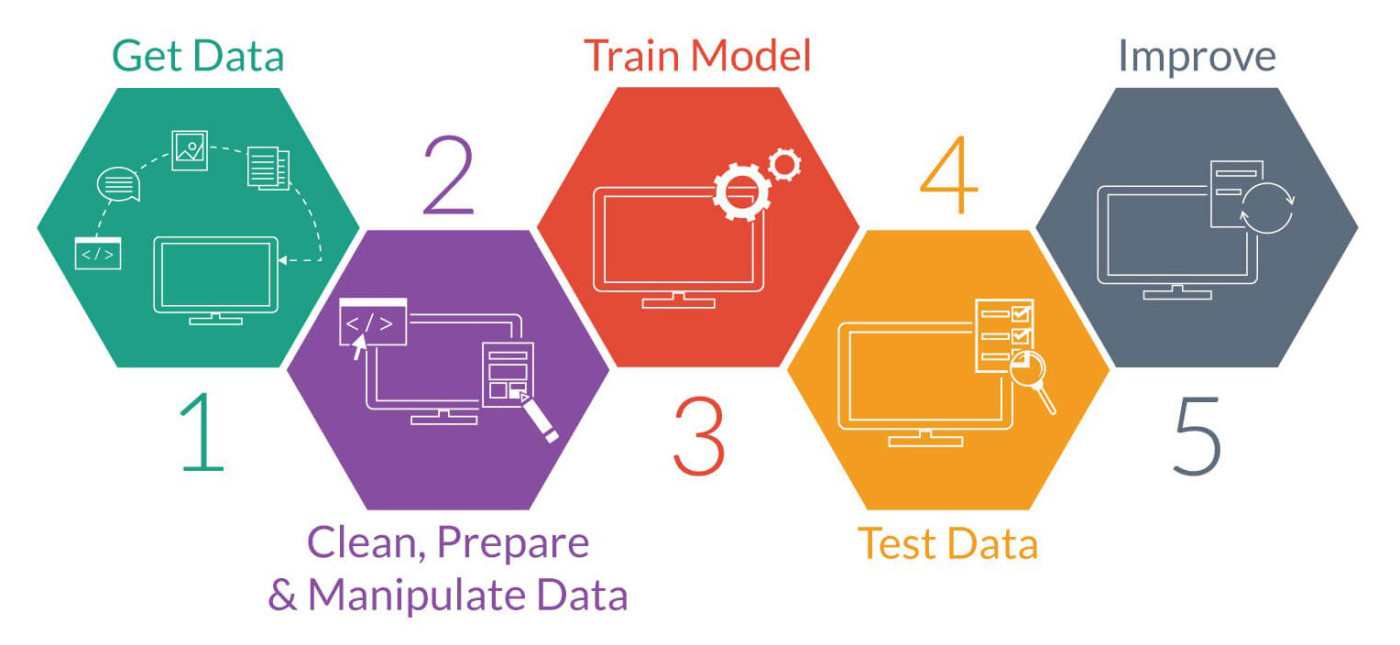# Intro

In its simplest form machine learning is using data to predict future events. There are two type of machine learning techniques ‘Supervised’ and ‘Unsupervised’

Supervised Algorithm: In supervised machine learning, a supervised learning algorithm analyzes the training data and produces an inferred function, which can be used for mapping new examples. An example of supervised ML can be SPAM detection software like SpamAssassin.

Unsupervised Algorithm: In unsupervised machine learing algorithms we are looking at cluster of like data. The algorithm analyses the input data and identifies groups of data that share traits.

Reinforcement learning:

## ML WorkFlow

A machine learning workflow is a orchestrated and repeatable pattern which systematically transforms and processes information to create prediction solutions.
Pointers for ML Workflow:
More data is better.
Later knowledge effects previous steps
Expect to go backwards.
Data is never as you need it.Tidy data : Tidy datasets are easy to manipulate, model and visualize, and have a specific structure (more like sql data). Cleaning and preparing data should produce tidy data. In python we can use panda’s dataframe to reformate the data.

Data correlation: It would be better for our models if the data is correlated as it can through off the model. If there are columns in a table that have one to one correlation then one of the column should be dropped.

Some Basic Algorithms
Each of these algorithms are classic machine learning algorithms.
Naive Bayes: This algorithm is based on Bayes theorem, predition is based on likelihood from previous data. It assumes that every feature has the same weight. Requires small amount of data to train.
Logistic Regression: In this algorithm relationship between features are weighted.
Decision Tree: The Decision tree alogrithm uses a binary tree where each node making a decision based on the values

ML Training is Letting specific data teach a Machine Learining algorithm to create a spcific prediction model and if the data changes we retrain.

## Jupyter NoteBook and Python for ML

One of the most significant advances in the scientific computing arena is underway with the explosion of interest in Jupyter (formerly, IPython) Notebook technology. There are now Jupyter Notebooks on numerous topics in many scientific disciplines.
It creates a single interactive document that contains text, code and even grahpics. It gives a great working environment for machine learning plaforms. It also gives a shared environment for teams of developers to work on. Languages supported by Jupyter includes python, Ruby, R, Haskell, C#, PHP and many others.
Installing Jupyter notebook with python:

``````#Make sure python3 and pip is installed
#Then update pip
python3 -m pip install --upgrade pip
#Install jupyter
python3 -m pip install jupyter
#To run the notebook type
jupyter notebook
``````

Installing required Libraries

``````pip install pandas
pip install matplotlib
pip install numpy
``````